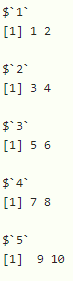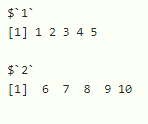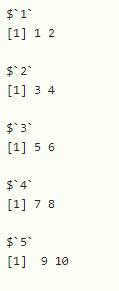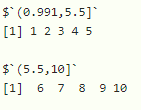# Split Vector into Chunks in R

• Last Updated : 23 Sep, 2021

In this article, we will discuss how to split vectors into chunks in R programming language.

## Method 1: By using the length of each chunk

In this scenario, a chuck length is specified, so that we can divide the vector using the split() function.

Syntax:

split(vector,   ceiling(seq_along(vector) / chunk_length))

where,

• vector is the input vector
• split() function is used to split the vector
• ceiling() is the function that takes two parameters one parameter that is vector with sequence along to divide the vector sequentially and second is chunklength, which represents the length of chunk to be divided

Example: R program to divide the vector into chunks with length

## R

 `# create a vector with 10 elements``vector=``c``(1,2,3,4,5,6,7,8,9,10)`` ` `# specify the chunk length as 2``chunklength=2`` ` `# split the vector by length``print``(``split``(vector,``ceiling``(``seq_along``(vector) / chunklength)))`

Output:Example: R program to divide the vector into chunks with length

## R

 `# create a vector with 10 elements``vector=``c``(1,2,3,4,5,6,7,8,9,10)`` ` `# specify the chunk length as 5``chunklength=5`` ` `# split the vector by length``print``(``split``(vector,``ceiling``(``seq_along``(vector) / chunklength)))`

Output:## Method 2: By using the number of chunks

In this scenario, the number of chunks is to be provided, so that we can divide the vector using the split() function.

Syntax:

split(vector,  cut(seq_along(vector), chunk_number, labels = FALSE)

where,

• vector is the input vector
• split() function is used to split the vector
• cut() is the function that takes three parameters one parameter that is a vector with sequence along to divide the vector sequentially, second is the chunk number that is for number of chunks to be divided and the last is for labels to specify the chunks range

Note:

• If the label is FALSE, it will not display the chunk size.
• If labels are not specified, it will display labels

Example: R program to divide the vector into chunks using chunk number

## R

 `# create a vector with 10 elements``vector=``c``(1,2,3,4,5,6,7,8,9,10)`` ` `# specify the chunk number as 5``chunk_no=5`` ` `# split the vector by chunk number by specifying ``# labels as FALSE``print``(``split``(vector, ``cut``(``seq_along``(vector),chunk_no,labels = ``FALSE``)))`

Output:Example: R program to divide the vector into chunks using chunk number

## R

 `# create a vector with 10 elements``vector=``c``(1,2,3,4,5,6,7,8,9,10)`` ` `# specify the chunk number as 2``chunkno=2`` ` `# split the vector by chunk number by ``# unspecifying labels ``print``(``split``(vector, ``cut``(``seq_along``(vector),chunkno)))`

Output:My Personal Notes arrow_drop_up